# MP Board Class 6th Maths Solutions Chapter 10 Mensuration Ex 10.3

## MP Board Class 6th Maths Solutions Chapter 10 Mensuration Ex 10.3

Question 1.
Find the areas of the rectangles whose sides are:
(a) 3 cm and 4 cm
(b) 12 m and 21m
(c) 2 km and 3 km
(d) 2 m and 70 cm
Solution:
(a) Area of rectangle = length × breadth
= 3 cm × 4 cm = 12 cm2

(b) Area of rectangle = length × breadth
= 12 m × 21 m = 252 m2

(c) Area of rectangle = length × breadth
= 2 km × 3 km = 6 km2

(d) Area of rectangle = length × breadth
= 2 m × 70 cm = 2 m × 0.7 m = 1.4 m2

Question 2.
Find the areas of the squares whose sides are:
(a) 10 cm
(b) 14 cm
(c) 5 m
Solution:
(a) Area of square = side × side
= 10 cm × 10 cm = 100 cm2

(b) Area of square = side × side
= 14 cm × 14 cm = 196 cm2

(c) Area of square = side × side
= 5 m × 5 m = 25 m2

Question 3.
The length and breadth of three rectangles are as given below:
(a) 9 m and 6 m
(b) 17 m and 3 m
(c) 4 m and 14 m
Which one has the largest area and which one has the smallest?
Solution:
(a) Area of rectangle = length × breadth
= 9m × 6m = 54m2

(b) Area of rectangle = length × breadth
= 17 m × 3 m = 51 m2

(c) Area of rectangle = length × breadth
= 4 m × 14 m = 56 m2
Thus, rectangle (c) has the largest area, i.e. 56 m2 and rectangle (b) has the smallest area, i.e., 51 m2.Question 4.
The area of a rectangular garden 50 m long is 300 sq m. Find the width of the garden.
SolutionL
Length of rectangle = 50 m
Area of rectangle = 300 m2
Since, area of rectangle = length × breadth
Therefore, breadth = $$\frac{\text { area of rectangle }}{\text { length }}$$
= $$\frac{300}{50}$$ m = 6 m
Thus, the breadth of the garden is 6 m.

Question 5.
What is the cost of tiling a rectangular plot of land 500 m long and 200 m wide at the rate of Rs. 8 per hundred sq m?
Solution:
Length of land = 500 m
Breadth of land = 200 m
Area of land = length × breadth
= 500 m × 200 m = 1,00,000 sq m
Cost of tiling 100 sq m of land = Rs. 8
∴ Cost of tiling 1,00,000 sq m of land
= Rs. $$\frac{8 \times 100000}{100}$$ = Rs. 8000

Question 6.
A table-top measures 2 m by 1 m 50 cm. What is its area in square metres?
Solution:
Length of table-top = 2 m
Breadth of table-top = 1 m 50 cm = 1.50 m
∴ Area of table-top = length × breadth
= 2 m × 1.50 m = 3 m2Question 7.
A room is 4 m long and 3 m 50 cm wide. How many square metres of carpet is needed to cover the floor of the room?
Solution:
Length of room = 4 m
And breadth of room = 3 m 50 cm = 3.50 m
∴ Area of carpet = length × breadth
= 4 m × 3.50 m = 14 m2

Question 8.
A floor is 5 m long and 4 m wide. A square carpet of sides 3 m is laid on the floor. Find the area of the floor that is not carpeted.
Solution:
Length of floor = 5 m
And breadth of floor = 4 m
Area of floor = length × breadth
= 5m × 4m = 20m2
Now, side of square carpet = 3 m
Area of square carpet = side × side
= 3m × 3m = 9m2
∴ Area of floor that is not carpeted
= 20 m2 – 9 m2 = 11 m2

Question 9.
Five square flower beds each of sides 1 m are dug on a piece of land 5 m long and 4 m wide. What is the area of the remaining part of the land?
Solution:
Side of square flower bed = 1 m Area of square flower bed = side × side
= 1m × 1m = 1m2
∴ Area of 5 square flower beds = (1 × 5) m2
= 5 m2
Now, length of land = 5 m
And breadth of land = 4 m
∴ Area of land = length × breadth = 5m × 4m
= 20 m2
∴ Area of remaining part
= Area of land – Area of 5 flower beds
= 20 m2 – 5 m2 = 15 m2Question 10.
By splitting the following figures into rectangles, find their areas (The measures are given in centimeters).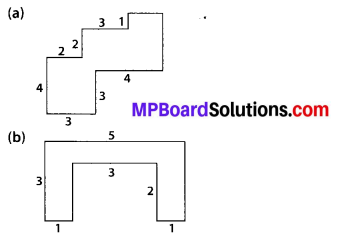Solution:
(a) We have,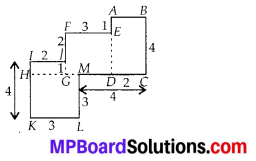Area of square HKLM = 3 × 3 cm2 = 9 cm2
Area of rectangle I]CH = 1 × 2 cm2 = 2 cm2
Area of square FEDG = 3 × 3 cm2 = 9 cm2
Area of rectangle ABCD = 2 × 4 cm2 = 8 cm2
∴ Total area of the figure = (9 + 2 + 9 + 8) cm2 = 28 cm2

(b) We have,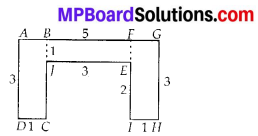Area of rectangle ABCD = 3 × 1 cm2 = 3 cm2
Area of rectangle BJEF = 3 × 1 cm2 = 3 cm2
Area of rectangle FGHI = 3 × 1 cm2 = 3 cm2
∴ Total area of the figure = (3 + 3 + 3) cm2 = 9 cm2

Question 11.
Split the following shapes into rectangles and find their areas. (The measures are given in centimetres).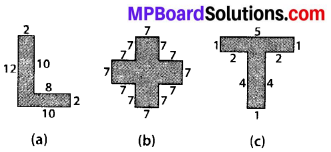Solution:
(a) We have,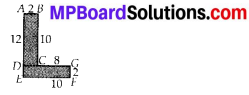Area of rectangle ABCD = 2 × 10 cm2 = 20 cm2
Area of rectangle DEFG = 10 × 2 cm2 = 20 cm2
∴ Total area of the figure = (20 + 20) cm2
= 40 cm2

(b) We have,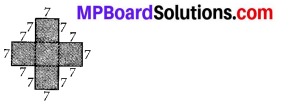There are 5 squares each of side 7 cm.
Area of one square = 7 × 7 cm2 = 49 cm2
∴ Area of 5 squares = 5 × 49 cm2 = 245 cm2

(c) We have,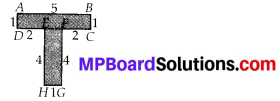Area of rectangle ABCD = 5 × 1 cm2 = 5 cm2
Area of rectangle EFGH = 4 × 1 cm2 = 4 cm2
∴ Total area of the figure = (5 + 4) cm2
= 9 cm2

Question 12.
How many tiles whose length and breadth are 12 cm and 5 cm respectively will be needed to fit in a rectangular region whose length and breadth are respectively:
(a) 100 cm and 144 cm
(b) 70 cm and 36 cm.
Solution:
(a) Area of rectangular region
= length × breadth = 100 cm × 144 cm = 14400 cm2
Area of one tile = 12 cm × 5 cm = 60 cm2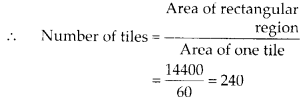Thus, 240 tiles are required.

(b) Area of rectangular region
= length × breadth = 70 cm × 36 cm = 2520 cm2
Area of one tile = 12 cm × 5 cm = 60 cm2
∴ Number of tiles
= $$\frac{\text { Area of rectangular region }}{\text { Area of one tile }}=\frac{2520}{60}$$ = 40
Thus, 42 tiles are required.